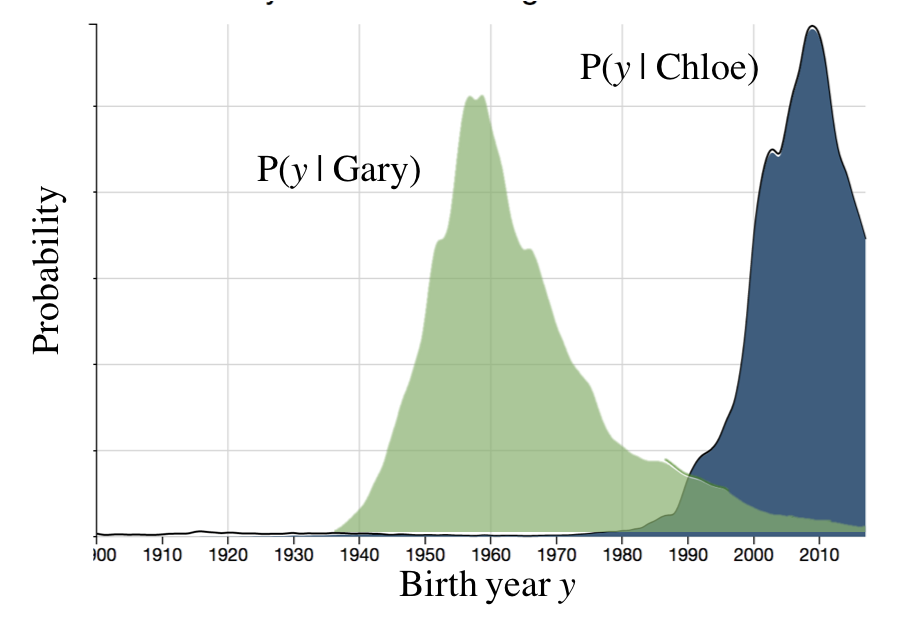Name to Age

Because of shifting patterns in name popularity, a person's age is a hint as to their age. The United States publishes a data which contains counts of how many US residents were born with a given name in a given year, based off Social Security applications. We can use inference to compute the reverse probability distribution: an updated belief in a person's age, given their name. As a reminder, if I know the year someone was born, I can calculate their age within one year.The US Social Security applications data provides you with a function: count(year, name) which returns the number of US citizens, born in a given year with a given name. You also have access to a list names which has each name ever given in the US and years which has all the years. This function is implicitly giving us the joint probability over names and birth year. The probability of a joint assignment to name and birth year can be estimated as the count of people with that name, born on that year, over the total number of people in the dataset. Let $B$ be the year someone is born, and let $N$ be their name: $$P(B = b,N = n) \approx \frac{\text{count}(b, n)} {\sum\limits_{i \in \text{names}} \sum\limits_{j \in \text{years}} \text{count}(i,j)}$$

The question we would really like to answer is: what is your belief that a resident was born in 1950, given that their name is Gary? We can get started by applying the definition of conditional probability for random variables: \begin{align*} \P( B = 1950 | N = \text{Gary} ) = \frac{\P(N = \text{Gary} , B = 1950)}{\P(N = \text{Gary})} \end{align*} But this leaves one term to compute: $\P(N = \text{Gary})$ which we can compute using marginalization: \begin{align*} \P( N = \text{Gary}) &= \sum_{y \in \text{years}} P(B = y,N = \text{Gary})\\ &\approx \frac{ \sum\limits_{y \in \text{years}} \text{count}(y, \text{Gary})}{\sum\limits_{i \in \text{names}} \sum\limits_{j \in \text{years}} \text{count}(i,j)} \end{align*}

Putting this all together we have: \begin{align*} \P( B = 1950 | N = \text{Gary} ) &= \frac{\P(N = \text{Gary} , B = 1950)}{\P(N = \text{Gary})} \\ &\approx \frac { \Big( \frac{\text{count}(1950, \text{Gary})} {\sum\limits_{i \in \text{names}} \sum\limits_{j \in \text{years}} \text{count}(i,j)} \Big) } { \Big( \frac{ \sum\limits_{y \in \text{years}} \text{count}(y, \text{Gary})}{\sum\limits_{i \in \text{names}} \sum\limits_{j \in \text{years}} \text{count}(i,j)} \Big) } \\ &\approx \frac { \text{count}(1950, \text{Gary}) } { \sum\limits_{y \in \text{years}} \text{count}(y, \text{Gary}) } \end{align*}

More generally, for any name, we can compute the conditional probability mass function over birth year $B$: \begin{align*} \P( B = b | N = n ) &\approx \frac { \text{count}(b, n) } { \sum\limits_{y \in \text{years}} \text{count}(y, n) } \end{align*}

From Birth Year to Age

Of course, if $B$ is the birth year of a person, their age, $A$ is approximately the current year minus $B$. This could be off by one if someone has a birth day later in the year, but we will ignore this small deviation for now. So for example, if we think that a person was born in 1988, since the current year is then their age is - 1988 =

Assumptions

This problem makes many assumptions which are worth highlighting. In fact, any time we make generalizations (especially about demographics) based on sparse information we should tread lightly. Here are the assumptions that I can think of:

1. This data only is accurate for names of people in the US. The probability of age given names could be very different in other countries.
2. The US census is not perfect. It does not capture all people who are resident in the US, and there are demographics which are underrepresented. This will also skew our results.

Demo

Query Name:

Records with name:

This demo is based on real data from US Social Security applications between 1914 and 2014. Thank you to https://www.kaggle.com/kaggle/us-baby-names for compiling the data. .

Names that Give Away Your Age

Some names have certain years where they were exceptionally popular. These names provide quite a lot of information about birth year. Let's look at some of the names with the highest max probability.

Medium Popularity (>10,000 people with the name)

NameAge with max probProb of most likely age
Katina 49 0.245
Marquita 38 0.233
Ashanti 19 0.250
Miley 13 0.250
Aria 7 0.247

High Popularity (>100,000 people with the name)

NameAge with max probProb of most likely age
Debbie 62 0.104
Whitney 35 0.098
Chelsea 29 0.103
Aidan 18 0.098
Some names don't seem to have enough data to make good probability estimates. Can we quantify our uncertainty in such probability estimates? For example, if a name has only 10,000 entries in the database, of which only 100 were born in the year 1950, how confident are we that the true probability for 1950 is $\frac{100}{10000} = 0.01$? One way to express our uncertainty would be through a Beta Distribution. In this scenario we could represent our belief in the probability for 1950 as $X \sim \Beta(a=101, b=9901)$ reflecting that we have seen 100 people born in 1950 and 9900 people who were not. We can plot that belief, zoomed into the range [0, 0.03]:
We can now ask questions such as, what is the probability that $X$ is within 0.002 of 0.01?
Semantically this leads to the claim that, after observing 100 births with a name in 1950, out of 10,000 births with that name over the whole dataset, there is a 95% chance that the probability of someone being born in 1950 is 0.010 $\pm$ 0.002.